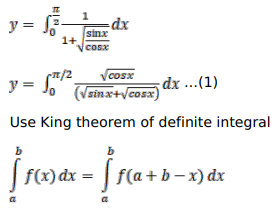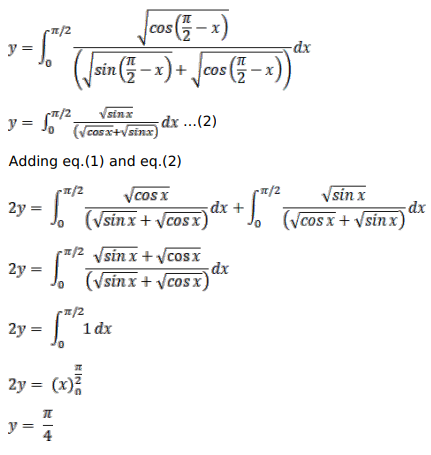# Solve this followingQuestion:

Prove that

$\int_{0}^{\pi / 2} \frac{d x}{(1+\sqrt{\tan x})}=\frac{\pi}{4}$

Solution: